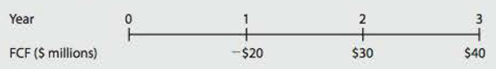Chapter 9, Problem 15PFundamentals of Financial Manageme...

14th Edition
Eugene F. Brigham + 1 other
ISBN: 9781285867977

Solutions

Chapter
SectionFundamentals of Financial Manageme...

14th Edition
Eugene F. Brigham + 1 other
ISBN: 9781285867977
Textbook Problem

CORPORATE VALUATION Dozier Corporation is a fast-growing supplier of office products. Analysts project the following free cash flows (FCFs) during the next 3 years, after which PCF is expected to grow at a constant 7% rate. Dozier’s WACC is 13%.a. What is Dozier’s horizon, or continuing, value? (Hint: Find the value of all free cash flows beyond Year 3 discounted back to Year 3.) b. What is the firm’s value today? c. Suppose Dozier has $100 million of debt and 10 million shares of stock outstanding. What is your estimate of the current price per share? a. Summary Introduction To compute: The horizon value for Company D. Corporate Valuation: The process to evaluate a firm on the basis of its future operations and the results of those operations is called corporate valuation. It is a useful tool to analyze a firm’s stock for investment purposes. Horizon Value: The value at a specified future date that includes the total of discounted amount to be received after that future date is called horizon value. The future date that is considered here, is the one after which the growth in cash flows will be constant forever. Explanation Given, Free cash flow at the end of third year is$40.

Constant growth rate is 7%.

WACC is 13%.

Formula to compute the horizon value,

Horizon Value=FCF3(1+g)WACCg

Where,

• FCF3 free cash flow at the end of 3rd year.
• g is constant growth rate of free cash flow.
• WACC is weighted average cost of capital

b.

Summary Introduction

To compute: The firm’s value for Company D.

Firm’s Value:

Also known as intrinsic value, it is the total of all present values of future free cash flows a company estimated from its operations. This is used as per the corporate valuation model to evaluate a firm.

c.

Summary Introduction

To compute: The current share price of Company D.

Still sussing out bartleby?

Check out a sample textbook solution.

See a sample solution

The Solution to Your Study Problems

Bartleby provides explanations to thousands of textbook problems written by our experts, many with advanced degrees!

Get Started

Find more solutions based on key concepts